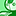Announcements
Product Release Webinar: Qlik Insider airing December 6! REGISTER TODAY!
cancel
Showing results for
Did you mean:Contributor

## If statement with variable won't work

Hello specialists,

I need help for a coding problem that I'm not understanding.

In my script I query the actual user with:

Let vBenutzer = QVUser();

Then I like to decide with a "IF" statement which text a second variable should have:

If(vBenutzer = 'XXX', 'EN', 'DE') as Language;

I have tried many versions of the "IF" statements but nothing works.

Please can every specialist give me support?

1 Solution

Accepted SolutionsMVP

Do you have section access in the application? If not, you might have to use OSUser() instead of qvuser(). And the code you use need some correction as well, like:

Let vBenutzer = QVUser();  // Or, OSUser()

Let Sprache =If( vBenutzer = 'XXX', 'EN', 'DE') ;

If Sprache = 'DE' then

SET A1 = 'Title DE 1';

SET A2 = 'Title DE 2';

end if;

If Sprache = 'EN' then

SET A1 = 'Title EN 1';

SET A2 = 'Title EN 2';

end if;

7 RepliesChampion III

Try this?

If( \$(vBenutzer) = 'XXX', 'EN', 'DE')Contributor
Author

Hello !

Sorry, but won't work. I receive the message "Error in Expression" if I change the statement to this:

If( \$(vBenutzer) = 'XXX', 'EN', 'DE') as Language;

The cursor indicates the " , " as the problem and I don't understand why?Creator IIIContributor
Author

Hello,

it's a short script until now. I like to check which user has opened the application. Then I like to decide in which

language should Qlikview shows the titles of the tables. But the script shows me an error in the first "if" statement

and indicates that the " , " should be the problem.

Let vBenutzer = QVUser();

If( \$(vBenutzer) = 'XXX', 'EN', 'DE') as Sprache;

If Sprache = 'DE' then

SET A1 = 'Title DE 1';

SET A2 = 'Title DE 2';

end if;

If Sprache = 'EN' then

SET A1 = 'Title EN 1';

SET A2 = 'Title EN 2';

end if;MVP

Do you have section access in the application? If not, you might have to use OSUser() instead of qvuser(). And the code you use need some correction as well, like:

Let vBenutzer = QVUser();  // Or, OSUser()

Let Sprache =If( vBenutzer = 'XXX', 'EN', 'DE') ;

If Sprache = 'DE' then

SET A1 = 'Title DE 1';

SET A2 = 'Title DE 2';

end if;

If Sprache = 'EN' then

SET A1 = 'Title EN 1';

SET A2 = 'Title EN 2';

end if;Contributor
Author

Thank you a lot, that was the solution !MVP & Luminary

In your case the variable contains a string and therefore you need single-quotes around it by calling them, like:

If('\$(vBenutzer)' = 'XXX', 'EN', 'DE')

Beside this I assume that you rather need osuser() instead of qvuser() which is only available if you used section access with USERID.

Further I suggest to consider to use the function directly instead of creating an additionally variable which adds here no value, maybe in this way:

if(match(osuser(), 'User1', 'User2', ...), 'EN', 'DE')

- MarcusCommunity Browser